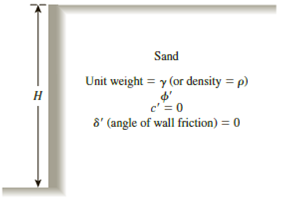Chapter 13, Problem 13.14PPrinciples of Geotechnical Enginee...

9th Edition
Braja M. Das + 1 other
ISBN: 9781305970939

Solutions

Chapter
SectionPrinciples of Geotechnical Enginee...

9th Edition
Braja M. Das + 1 other
ISBN: 9781305970939
Textbook Problem

13.13 through 13.16 Assume that the retaining wall shown in Figure 13.39 is frictionless. For each problem, determine the Rankine passive force per unit length of the wall, the variation of active earth pressure with depth, and the location of the resultant.Figure 13.39

To determine

The Rankine passive force σp(z=H) per unit length of the wall, the variation of active earth pressure Pa with depth, and the location z¯ of the resultant measured from the bottom of the wall.

Explanation

Given information:

The height (H) of the retaining wall is 25 ft.

The soil friction angle ϕ is 33°.

The angle of wall friction δ is 0.

The unit weight γ of the soil is 119lb/ft3

Calculation:

Calculate the passive earth pressure coefficient (Kp) using the relation.

Kp=tan2(45+ϕ2)

Substitute 33° for ϕ.

Ka=tan2(45+33°2)=tan2(45+16.5)=tan2(61.5)=3.392

Calculate Rankine passive force σp(z=H) per unit length of the wall using the relation.

σp(z=H)=KpγH

Substitute 3.392 for Kp, 119lb/ft3 for γ, and 25 ft for H.

σp(z=H)=3.392×119×25=10,091lb/ft2

Thus, the Rankine passive force per unit length of the wall is 10,091lb/ft2_

Still sussing out bartleby?

Check out a sample textbook solution.

See a sample solution

The Solution to Your Study Problems

Bartleby provides explanations to thousands of textbook problems written by our experts, many with advanced degrees!

Get Started

Find more solutions based on key concepts